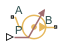# Pressure Source (TL)

Generate constant pressure differential

• Library:
• Simscape / Foundation Library / Thermal Liquid / Sources

•## Description

The Pressure Source (TL) block represents an ideal mechanical energy source in a thermal liquid network. The source can maintain the specified pressure differential across its ports regardless of the mass flow rate through the source. There is no flow resistance and no heat exchange with the environment. A positive pressure differential causes the pressure at port B to be greater than the pressure at port A.

The energy balance at the source is a function of the energy flow rates through ports A and B and the work done on the fluid:

`${\varphi }_{A}+{\varphi }_{B}+{\varphi }_{work}=0,$`

where:

• ϕA is the energy flow rate into the source through port A.

• ϕB is the energy flow rate into the source through port B.

• ϕwork is the isentropic work done on the fluid.

The isentropic work term is

`${\varphi }_{work}=\frac{\stackrel{˙}{m}\left({p}_{B}-{p}_{A}\right)}{{\rho }_{avg}},$`

where:

• ϕwork is the isentropic work done on the thermal liquid.

• pA is the pressure at port A.

• pB is the pressure at port B.

• ρavg is the average liquid density,

`${\rho }_{avg}=\frac{{\rho }_{A}+{\rho }_{B}}{2}\text{.}$`

### Assumptions and Limitations

• There are no irreversible losses.

• There is no heat exchange with the environment.

## Ports

### Conserving

expand all

Thermal liquid conserving port. A positive pressure differential causes the pressure at port B to be greater than the pressure at port A.

Thermal liquid conserving port. A positive pressure differential causes the pressure at port B to be greater than the pressure at port A.

## Parameters

expand all

Desired pressure differential across the ports of the source.

Area normal to the direction of flow at the source inlet and outlet. The two cross-sectional areas are assumed identical.

## Version History

Introduced in R2013b